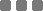## Friday, March 11, 2016

### 1. List of Numbers to List of Strings

For example, we have this object:

In JavaScript, it's called `Array` and in Python it's a `List`.

And for some reason, we need to convert every member into `String`. As you can see above, every member is a number (integer).

#### A. JavaScript

We can use the new method `map()` to do that. Basic looping method using `for`, `while`, and `do - while` can also do the task.

But of course, because `map()` is designed to be sweeter than the basic methods, let's use it.

And BYAM!
Variable `b` now consists of all members of variable `a`, but each of them is now a string.

Use your browser console, and copy paste the code above. And then put `console.log(b);` to see its content.

#### B. Python

In Python, when we design some structure which needs the conversion for some other process, we can use this shorthand:

As in:

That's it!

Well, not really.

You can use Python interpreter (command line) to `print` the variable `b` (the result).
It will have the same members as variable `a`, but every (number) member is converted into a string.

To make it "safer", use this other shorthand to substitute the `b` variable method:

Hm?
• The `str()` is to convert anything to string.
• The `v` is each member of variable `a`.
• The `a` is the input variable (list) we wanna convert.
• And the square brackets, `[...]`, are to initiate a `list` object.

### 2. The Other Way Round

#### A. JavaScript

For instance, we wanna extract the number only from an array which has that.

Let's use this object example:

It has string and number. Let's implement again the `map()` method.

And variable `b` now is the number part of every variable `a`'s member.

Wut?

• The `toString()` method is needed so when the method meets a non-string element, particularly the `match` method, it will have no problem for searching using `RegExp`.
• The `match()` is the `RegExp` searcher, with the `\d+` pattern.
The `\d` is the shorthand for digit (number). We can also use `[0-9]`.
The plus sign `+` is for pattern search for number that has 1 digit length or more.
The `g` flag is for searching globally within the string.
• And the `` after the `match()` method means that we're only interested in the first finding[?] (index 0).
[?]The `match()` method produces array of found patterns.

#### B. Python

Let's use the same input. And activate Python power.

On the above example, the list is converted into string, by using `str(a)`. Then `findall()` transformed that string into list (of found patterns). And then the string to number (integer) mapping begins -- the `map()` function.

For that kind of input, which has digit pattern in every string (and just number), the method will produce the exact same output as the JavaScript version.

But, for scattered around number, e.g. the number patterns for each member is separated by non-digit, and we just wanna take the first found number pattern (index 0), we can substitute the method for variable `b` into this:

Or this:

Whichever the most proper one.

But, if we time the execution using `timeit` module, the `map()` shorthand is faster than the second one. The populating list using that `for in` method. The third snippet is the slowest, since it's just the second one but then it's mapped. Like, seriously.

Anywho, be careful if you then have list member which has no digit in it -- because of the `findall()` method.
Put some additional filter before using any of those snippets above if you know that your input is gonna have mixed elements.
Or use the standard looping.

.......

...

I'll be going now. Thanks for visiting.JavaScript (and Python): Mapping Array/List of Numbers to Strings and the Other Way Round
https://monkeyraptor.johanpaul.net/2016/03/javascript-and-python-mapping-arraylist.html